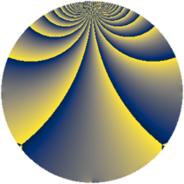# Properties

 Label 121.2.gLevel $121$ Weight $2$ Character orbit 121.g Rep. character $\chi_{121}(4,\cdot)$ Character field $\Q(\zeta_{55})$ Dimension $400$ Newform subspaces $1$ Sturm bound $22$ Trace bound $0$

# Related objects

## Defining parameters

 Level: $$N$$ $$=$$ $$121 = 11^{2}$$ Weight: $$k$$ $$=$$ $$2$$ Character orbit: $$[\chi]$$ $$=$$ 121.g (of order $$55$$ and degree $$40$$) Character conductor: $$\operatorname{cond}(\chi)$$ $$=$$ $$121$$ Character field: $$\Q(\zeta_{55})$$ Newform subspaces: $$1$$ Sturm bound: $$22$$ Trace bound: $$0$$

## Dimensions

The following table gives the dimensions of various subspaces of $$M_{2}(121, [\chi])$$.

Total New Old
Modular forms 480 480 0
Cusp forms 400 400 0
Eisenstein series 80 80 0

## Trace form

 $$400q - 44q^{2} - 32q^{3} - 34q^{4} - 43q^{5} - 22q^{6} - 44q^{7} - 44q^{8} - 110q^{9} + O(q^{10})$$ $$400q - 44q^{2} - 32q^{3} - 34q^{4} - 43q^{5} - 22q^{6} - 44q^{7} - 44q^{8} - 110q^{9} - 22q^{10} - 33q^{11} + 6q^{12} - 11q^{13} - 18q^{14} + 16q^{15} - 30q^{16} - 44q^{17} + 11q^{18} - 44q^{19} - 36q^{20} - 11q^{21} - 34q^{23} + 77q^{24} - 31q^{25} - 38q^{26} + 40q^{27} - 44q^{28} - 44q^{29} - 11q^{30} - 17q^{31} - 44q^{32} - 11q^{33} - 76q^{34} - 44q^{35} + 57q^{36} - 4q^{37} + 34q^{38} - 11q^{39} + 33q^{40} - 44q^{41} + 3q^{42} - 44q^{43} - 33q^{44} - 12q^{45} - 44q^{46} - 36q^{47} + 9q^{48} + 16q^{49} + 88q^{50} + 154q^{51} + 110q^{52} + 78q^{53} + 231q^{54} + 44q^{55} - 55q^{56} + 66q^{57} + 73q^{58} - 33q^{59} + 15q^{60} - 44q^{61} + 11q^{62} + 121q^{63} + 96q^{64} - 11q^{65} - 41q^{67} - 44q^{68} + 8q^{69} + 24q^{70} + 15q^{71} + 77q^{72} - 22q^{73} - 44q^{74} + 7q^{75} + 176q^{76} + 88q^{77} - 21q^{78} + 44q^{79} + 171q^{80} + 37q^{81} + 22q^{82} - 44q^{83} - 11q^{84} + 66q^{85} - 20q^{86} - 11q^{87} + 77q^{88} + 3q^{89} + 264q^{90} + 79q^{91} + 228q^{92} + 49q^{93} + 143q^{94} + 44q^{95} + 121q^{96} + 10q^{97} + 132q^{98} + 143q^{99} + O(q^{100})$$

## Decomposition of $$S_{2}^{\mathrm{new}}(121, [\chi])$$ into newform subspaces

Label Dim. $$A$$ Field CM Traces $q$-expansion
$$a_2$$ $$a_3$$ $$a_5$$ $$a_7$$
121.2.g.a $$400$$ $$0.966$$ None $$-44$$ $$-32$$ $$-43$$ $$-44$$